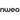# 4 Easy Ways for Multiplying Exponents [+ Activities]

All PostsWhat do earthquakes, the stock market, computer science and nuclear physics all have in common?

They all involve multiplying exponents.

Exponents are an essential part of algebra, polynomial equations and higher-level math courses, but many students struggle to understand how to work with them. You’ve gone through exponent rules with your class, and now it’s time to put them in action.

## Let’s review: Exponent rulesBefore you start teaching your students how to multiply exponents, you might want to do a quick review with them on the basics of how exponents work.

Exponents (also called powers) are governed by rules, like everything else in math class. Here’s a quick recap:

An exponent is a way of expressing repeated multiplication. For example, 35 represents three multiplied by itself five times:

35 = 3 × 3 × 3 × 3 × 3 = 243

35 = 243

The first number is referred to as the base. It represents the number that gets multiplied.

The second, smaller number is the exponent. It represents the number of times the base is multiplied by itself.

There are seven exponent rules:

1. Product of powers rule: Add powers together when multiplying like bases
2. Quotient of powers rule: Subtract powers when dividing like bases
3. Power of powers rule: Multiply powers together when raising a power by another exponent
4. Power of a product rule: Distribute power to each base when raising several variables by a power
5. Power of a quotient rule: Distribute power to all values in a quotient
6. Zero power rule: Any base raised to the power of zero becomes one
7. Negative exponent rule: To change a negative exponent to a positive one, flip it into a reciprocal

Got it? Then let’s keep going.

## How to multiply exponents 4 ways

Remember, all these strategies are just shortcuts to help simplify more complicated equations. In order to find the actual value of an exponent, students must first understand what it means: repeated multiplication.

Get students familiar with the basics, like expressing exponents as products, before you move on to multiplying exponents.

When they’re comfortable with the concept, it’s time to get started.

### 1. Multiplying exponents with the same base

When you’re multiplying exponents, use the first rule: add powers together when multiplying like bases.

52 × 56 = ?

The bases of the equation stay the same, and the values of the exponents get added together.

52 × 56 = 58

But why does it work? Let’s look a little closer: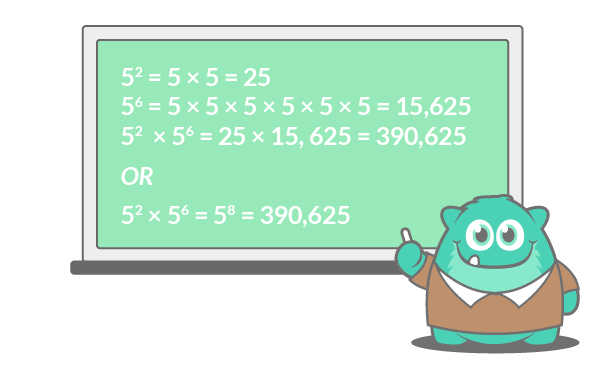Adding the exponents together is just a shortcut to the answer. When we add the exponents, we’re increasing the number of times the base is multiplied by itself.

This rule stays the same, no matter how complicated the question gets. Here’s a trickier example with variables:

(2𝒙8)(3𝒙5) = ?

First, multiply the numbers (2 and 3) together since they’re coefficients, not the base. (A coefficient is a number multiplied by a variable like 𝒙.)

(2𝒙8)(3𝒙5) = 6𝒙13

### 2. Multiplying exponents with different bases

It is possible to multiply exponents with different bases, but there’s one important catch: the exponents have to be the same.

Here’s how you do it:

54 × 24 = ?

First, multiply the bases together. Then, add the exponent. Instead of adding the two exponents together, keep it the same.

54 × 24  = 104

This is why it works: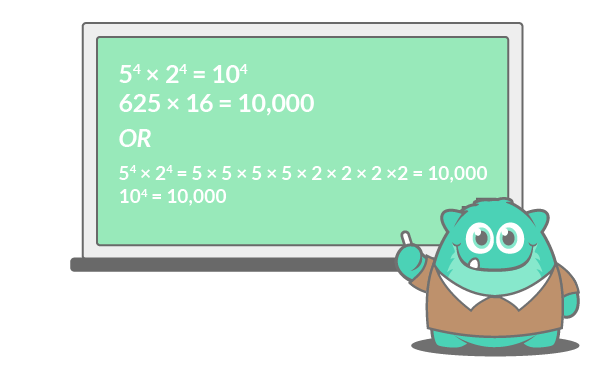This is because of the fourth exponent rule: distribute power to each base when raising several variables by a power. This equation can also be written as (5×2)4, meaning that the exponent is distributed to the 5 and 2.

Now, let’s try multiplying variables with exponents.

(3y3)(4y3) = ?

Remember, the rule holds true as long as the exponents and the variables are the same (because 𝒙 and y variables can’t be combined).

(3y3)(4y3) =12y3

### 3. Multiplying exponents with different bases and exponents

What happens when you want to multiply different exponents with different bases?

The short answer is that you can’t. Unlike the examples above, there’s no shortcut.

For example: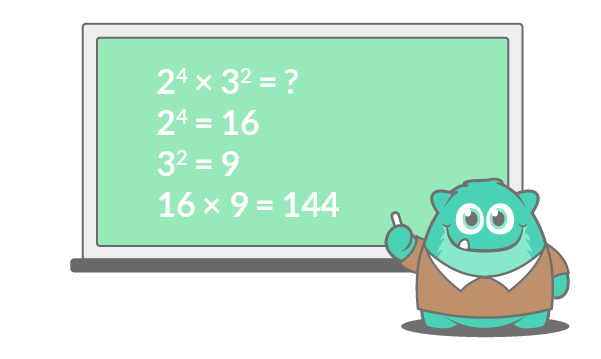Since 24 and 32 have nothing in common to combine, the answer can’t be simplified down to a single exponent and must be expressed as a regular number.

### 4. Multiplying negative exponents

It might seem complicated, but multiplying exponents with negative numbers is exactly the same as multiplying exponents with non-negative numbers.

Begin with reviewing the properties of negative numbers. Specifically, review how to add and multiply them. Your students need to feel comfortable working with negative numbers before they move on to negative exponents.

Then, remember the seventh exponent rule: to change a negative exponent to a positive one, flip it into a reciprocal.

#### Same base, different exponents:

4-3 × 42 = ?

Remember — add exponents with like bases.

4-3 × 42 = 4-1

To solve this exponent, flip the negative exponent into a reciprocal.

4-1 = ¼= 0.25

#### Different base, but the same exponents:

2-5 × 3-5 = ?

Just like above, multiply the bases and leave the exponents the same.

2-5 × 3-5 = 6-5

To solve, flip the negative exponent into a reciprocal.

6-5 = ⅙5

#### If the exponents have nothing in common, solve the equation directly:

2-3 × 32

First, flip the negative exponents into reciprocals, then calculate.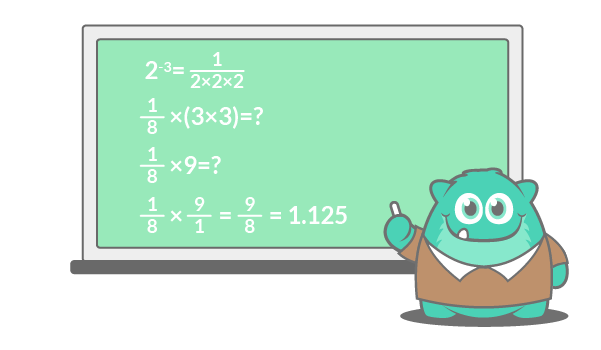When you’re multiplying exponents, remind students to:

• Add the exponents if the bases are the same
• Multiply the bases if the exponents are the same
• If nothing’s the same, just solve it

## Activities for practicing multiplying exponents

### 1. Prodigy

Building math fluency is an important part of making sure students feel confident in high school- and college-level math courses. Students can practice multiplying exponents and other math concepts with Prodigy, while you deliver customized in-game questions based on lesson content.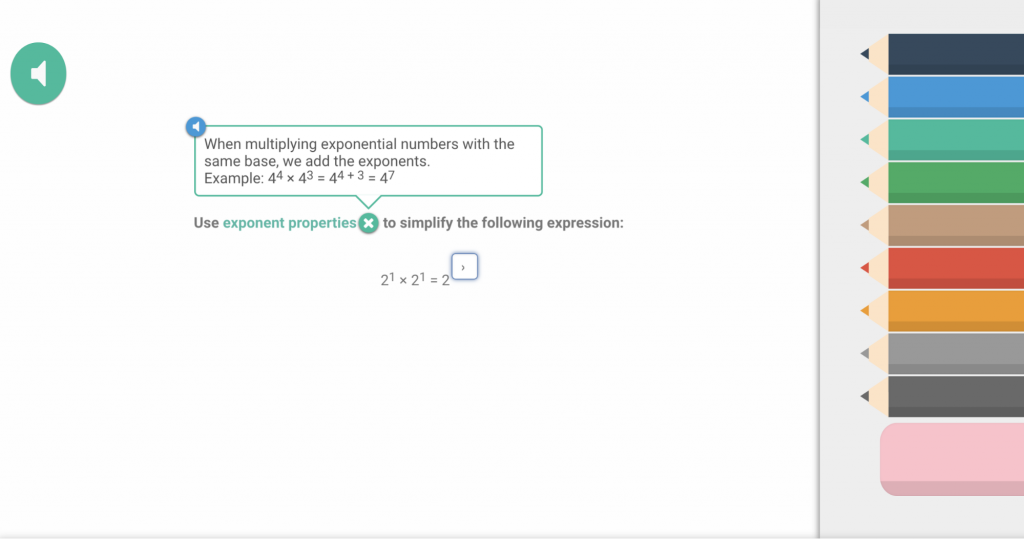Your class will explore a world filled with exciting quests, exotic pets and math learning. You’ll be able to choose what questions they answer and get real-time data about what they’ve mastered, what they’re working on and where they might need extra help.

With 1,400 skills and counting, you’ll be able to deliver curriculum-aligned content on any topic you’re covering, including multiplying exponents.### 2. Exponent WarThe classic card game — but with an exponentially fun twist!

Students work in teams of two and face off against another pair. Give each team a deck of cards (with the queens, jacks, and kings taken out) and have each player pull out two cards. The first card is the base, and the second card is the exponent.

Each pair has to race to solve their equation and find the product. The team with the highest answer wins. Set a timer for the class and see who can rack up the most points.

While students are playing, walk around the classroom and make sure they’re not missing any steps. If you see a lot of mistakes or struggling students, take it as a sign that you might need to do some review.

### 3. Exponent Scavenger Hunt

Give your students a chance to search for treasure and explore the classroom with an exponent scavenger hunt.

Divide your class into groups of three or four. Depending on the number of groups you have, make several different sets of cards. Start each set with a card that has a problem on it. Write the answer to the problem on the next card, and put another problem on the back. Keep going until you have three or four sets of problems (or more).

Starting with the first card, each group must solve the problem and find the correct answer somewhere else in the classroom. When they find the correct answer card, they can flip it over and solve the next problem. Give students scrap paper for solving, and let them start hunting for their answers. Whichever team finishes first is the winner!

### 4. Exponent JeopardyEvery student loves a classic game of Jeopardy. Using a customizable template, replace the trivia with questions that give students a chance to practice multiplying exponents, and divide the class into two teams.

Here are some tips to make sure the game runs smoothly:

• If you have a larger class, consider dividing the classroom into more than one game so every student has a chance to participate
• To combine math and computer skills, challenge students to make the game themselves. Give them the template (or have more advanced students start from scratch) and ask them to make a short game.
• Use it as an end-of-unit review activity before a test, and combine higher-value questions with more complicated answers

### 5. Worksheets for multiplying exponents

Worksheets are a tried-and-true method for developing math fluency in a particular set of skills. They can also be an indicator of student understanding when used as part of a formative assessment strategy. Here are some of our favorites:

If you’re looking for a worksheet that covers more than just multiplying exponents, check out our exponent rules worksheet (with answer key).

For something more unique, try this multiplying polynomials activity. Like a regular worksheet, it has questions for students to answer, but it also contains an “answer bank” for students. Cut out the accompanying strips and mix them up. Have students match answers to the correct section on their worksheet after solving the equation and showing their work.

## Multiplying exponents: Let’s review

If there are only three things your students remember, make sure it’s these concepts:

• Add powers together when multiplying like bases
• Multiply bases when multiplying like exponents
• Exponents are the product of repeated multiplication

If they remember those three rules, they’ll have a solid foundation built even before their first high school algebra class.

As always, take it slow and make sure students understand the basics before things get more complicated. It might seem like a complex idea to teach, but stick to the steps and progress in a logical order to see your students’ knowledge grow.

Create or log into your teacher account on Prodigy— a free, game-based learning platform for math that’s easy to use for educators and students alike. Aligned with curricula across the English-speaking world, it’s loved by more than a million teachers and 50 million students.
•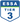•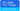•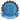•